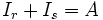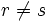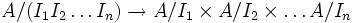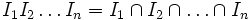# Chinese remainder theorem

(diff) ← Older revision | Latest revision (diff) | Newer revision → (diff)
Suppose$I_1, I_2, \ldots, I_n$ are ideals in a commutative unital ring$A$, with the property that any two of them are comaximal; in other words,$I_r + I_s = A$ for$r \ne s$. Then the natural map below is an isomorphism:$A/(I_1I_2 \ldots I_n) \to A/I_1 \times A/I_2 \times \ldots A/I_n$$I_1I_2\ldots I_n = I_1 \cap I_2 \cap \ldots \cap I_n$